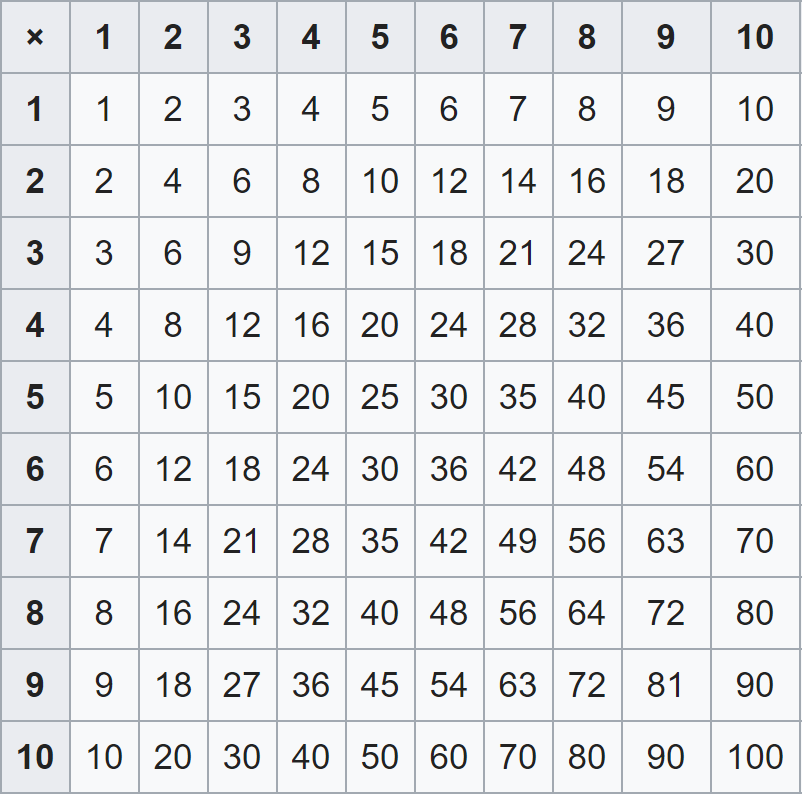# Space Complexity

We can also look at a program based on the amount of space, or memory, that it uses. In this case, we are looking at the number of variables that are needed, and also the size of any lists, arrays, or more advanced data structures used.

The comparison in terms of space leads us to observe that the linear solution uses, in addition to the input value `x` for each iteration of the loop, also the `max` and `min` variables. So, there are just 3 more variables to keep track of.

The pairs solution uses, in addition to the two input values `x` and `y` for each iteration of the loop, two variables for the `lastmax` and `lastmin` as well as the global `max` and `min` variables. So, there are 6 more variables to keep track of in this solution

Therefore, the linear solution is more space-efficient since it requires only three variables.

## A Bigger Example

Let’s consider a bigger example program, just to see the impact of space complexity when analyzing a program. Consider a program that will compute the result of multiplying two numbers from \$1\$ through \$10\$. So, there are 10 numbers we need to consider as both operands.

One possible solution would be to pre-compute all possible answers in a 10 by 10 array, which would contain 100 elements. A diagram of this is shown below.^[Wikipedia contributors. (2020, January 25). Multiplication table. In Wikipedia, The Free Encyclopedia. Retrieved 02:06, January 28, 2020, from https://en.wikipedia.org/w/index.php?title=Multiplication_table&oldid=937470066]

This is a very inefficient program in terms of space complexity, since it requires \$N^2\$ spaces in memory for \$N\$ possible operand values.

Of course, we already know that we could write a program that could simply calculate and return the answer based on the input values, so this example is a bit worthless in practice. However, on some small, embedded systems such as a smart watch or nano-machine, we might discover that it is better to use memory than to spend time calculating a result on such a slow processor, so there are times where having higher space complexity helps save time in the long run.